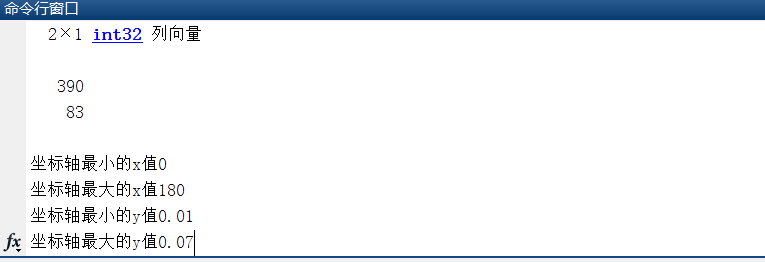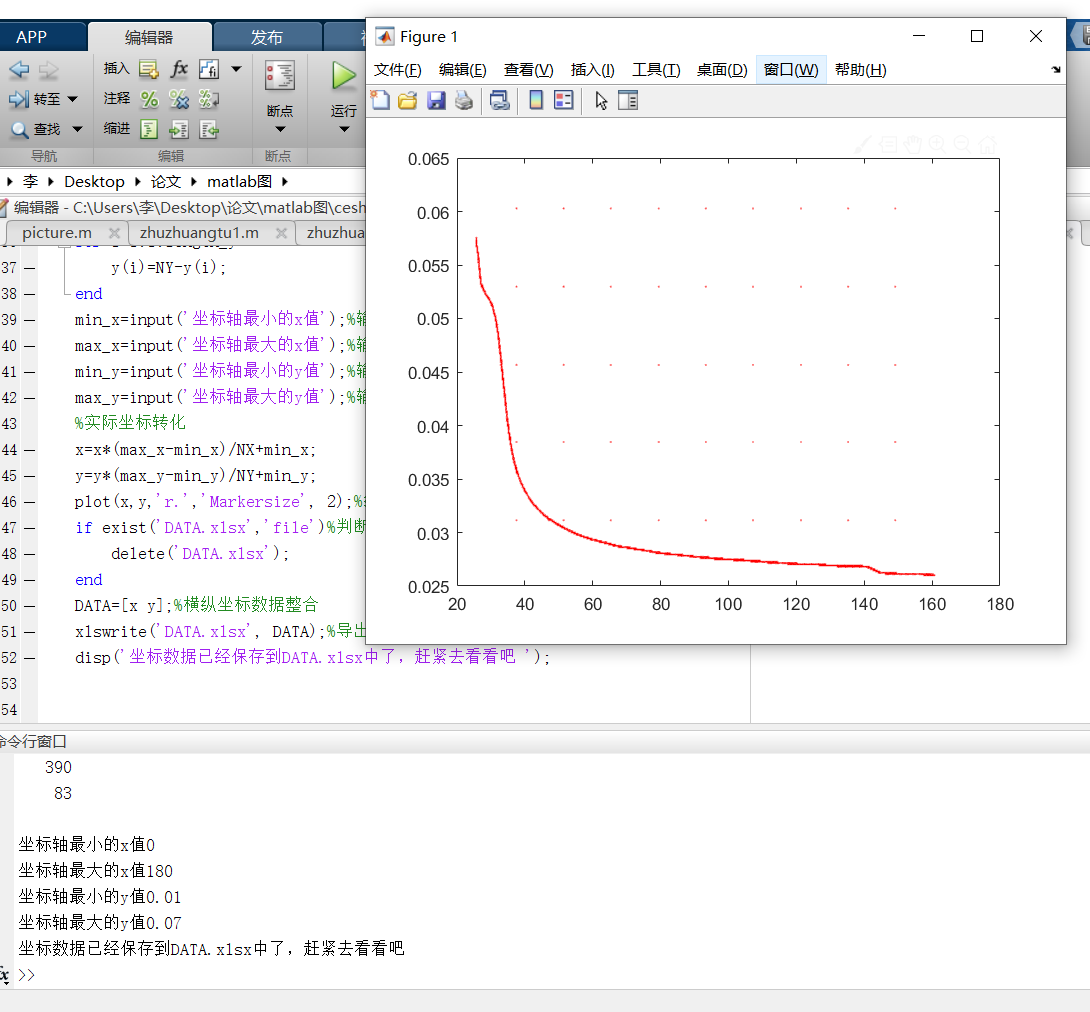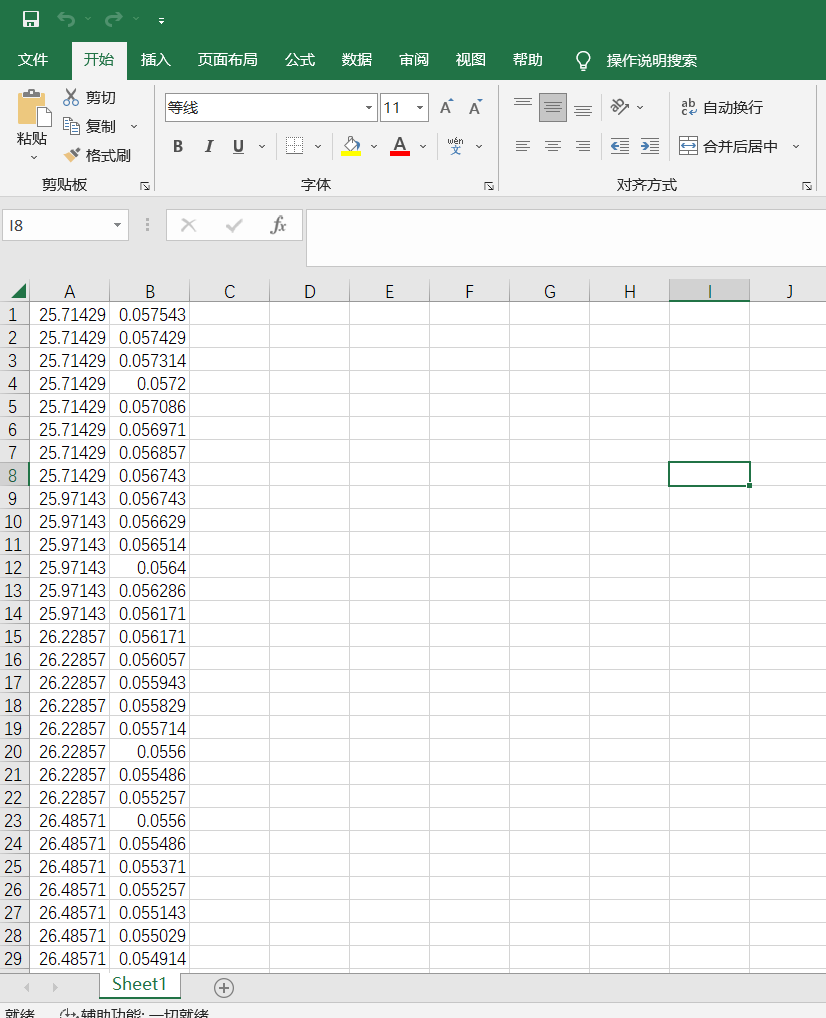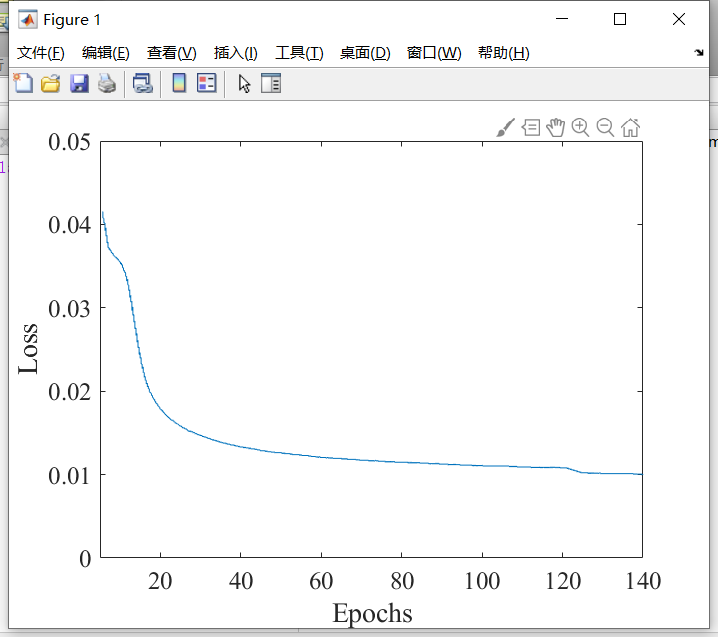# matlab复现论文中的曲线图（坐标存入excal，然后导入matlab画图）clc,clear;disp(‘提示：命令行窗口键入：ctrl+c，表示停止运行 ‘);im=imread(‘1.jpg’);%读入图片(替换成需要提取曲线的图片)im=rgb2gray(im);%灰度变化thresh = graythresh(im);%二值化阈值thresh=thresh+0.2;%有时候thresh的值偏低，可以手动调节提高一点，这里就提高了im=im2bw(im,thresh);%二值化set(0,’defaultfigurecolor’,’w’);%设置MATLAB中figure的背景为白色disp(‘请在Figrure中先后点击图片窗口,先左下取一点，然后右上取一点,用于裁剪掉坐标轴的信息 ‘);input(‘理解OK了之后输入”1”即可开始’);imshow(im)%显示图片[NY,NX]=size(im);%获取图片像素矩阵的大小[XX,YY]=ginput(2);%——获取图片剪裁的地方，消除坐标轴，先左下取一点，然后右上取一点%把数据转换为整型XX=int32(XX)YY=int32(YY)%消除坐标轴，让其变成白色for i=1:1:XX(1) im(:,i)=1;endfor i=XX(2):1:NX im(:,i)=1;endfor i=1:1:YY(2) im(i,:)=1;endfor i=YY(1):1:NY im(i,:)=1;endimshow(im)%显示图片pause(1);%暂停一下，看看裁剪效果close();%可以了，不看了[y,x]=find(im==0);%找出图形中的“黑点”的坐标。该坐标是一维数据。%坐标转换,变换成正常坐标轴length_y=length(y);for i=1:1:length_y y(i)=NY-y(i);endmin_x=input(‘坐标轴最小的x值’);%输入x轴最小值max_x=input(‘坐标轴最大的x值’);%输入x轴最大值min_y=input(‘坐标轴最小的y值’);%输入y轴最小值max_y=input(‘坐标轴最大的y值’);%输入y轴最大值%实际坐标转化x=x*(max_x-min_x)/NX+min_x;y=y*(max_y-min_y)/NY+min_y;plot(x,y,’r.’,’Markersize’, 2);%绘制最终坐标图if exist(‘DATA.xlsx’,’file’)%判断存储数据的文件是不是存在，存在就删掉 delete(‘DATA.xlsx’);endDATA=[x y];%横纵坐标数据整合xlswrite(‘DATA.xlsx’, DATA);%导出到exceldisp(‘坐标数据已经保存到DATA.xlsx中了，赶紧去看看吧 ‘);data=xlsread(‘C:\Users\李\Desktop\论文\matlab图\DATA.xlsx’) x=data(:,1); %第1列的全部y=data(:,2); %第2列的全部plot(x,y) ; %绘制曲线axis([5 140 0 0.06])set(gca,’fontsize’,15,’fontname’,’Times’);xlabel(‘Epochs’)ylabel(‘Loss’)